Vibroengineering PROCEDIA

Published: 07 May 2020

# Optimal design parameters of drum’s isolation system for a double-drum vibratory roller

Le Van Quynh1
Vi Thi Phuong Thao2
Truong Tu Phong3
1Faculty of Automotive and Power Machinery Engineering, Thai Nguyen University of Technology, Thai Nguyen, Vietnam
2Faculty of International Training, Thai Nguyen University of Technology, Thai Nguyen, Vietnam
3Center for Technical Practice, Thai Nguyen University of Technology, Thai Nguyen, Vietnam
Corresponding Author:
Le Van Quynh
Views 175

#### Abstract

The aim of this study is to propose an optimal design method for drum’s isolation systems of a double-drum vibrating roller so that the ride comfort can be improved. A half-vehicle ride dynamic model is established based on the drum-ground interactions. The weighted root mean square (rms) of acceleration responses of the vertical and pitch vibrations of the vehicle body (${a}_{wb}$ and ${a}_{wphi}$) according to the ISO 2631:1997(E) standard is chosen as objective functions. A genetic algorithm (GA) is applied for searching for the optimal design parameters of drum’s isolation systems based on the objective functions. The study results indicate that the ${a}_{wb}$ and ${a}_{wphi}$ values of GA optimal parameters reduce significantly in comparison with those of the original parameters of drum’s isolation systems, which means that the performance optimization of drum’s isolation systems is better than the original vehicle in improving the vehicle ride comfort.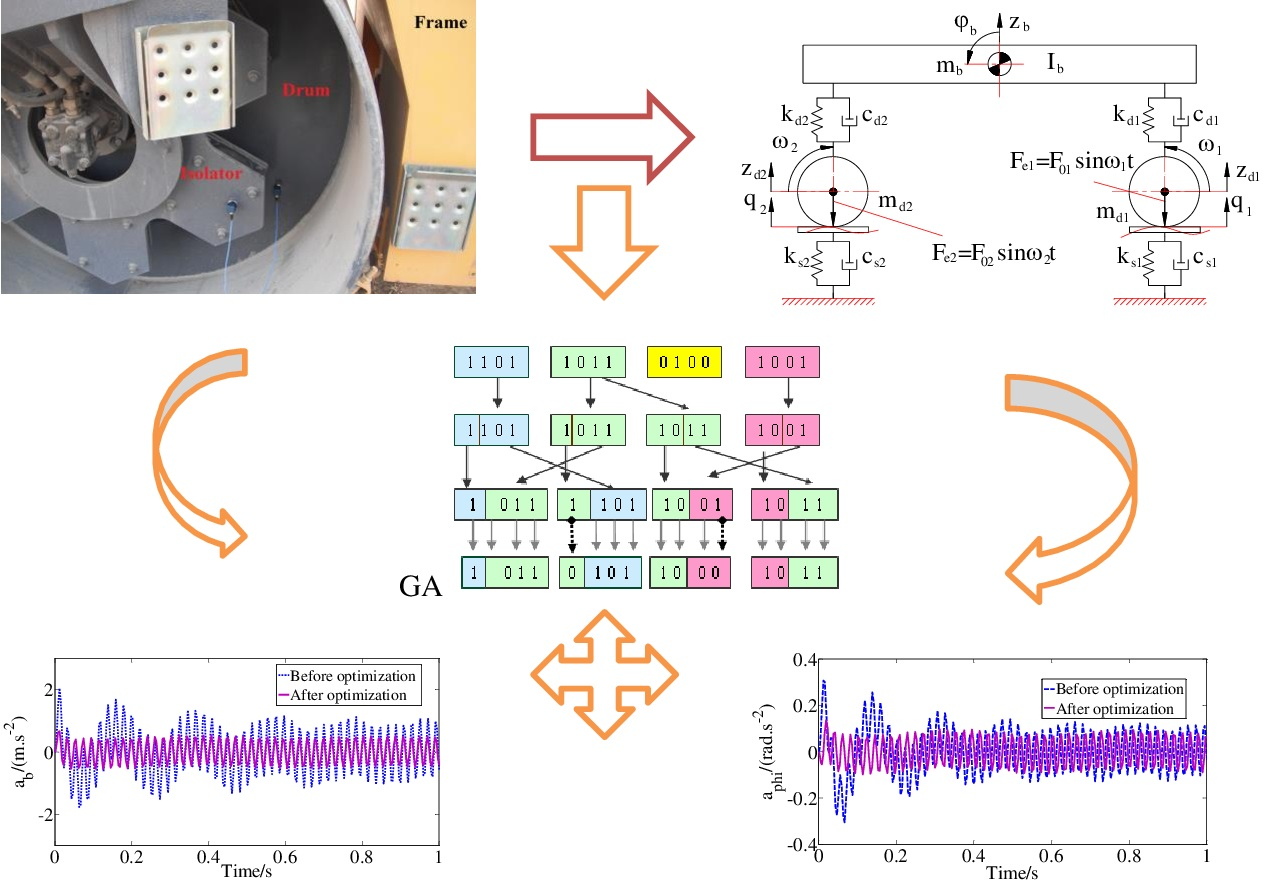#### Highlights

• The optimal design method for drum’s isolation systems of a double-drum vibrating roller is proposed in this study.
• The genetic algorithm (GA) is applied for searching for the optimal design parameters of drum’s isolation systems.
• The performance optimization of drum’s isolation systems is better than the original vehicle in improving the vehicle ride comfort.

## 1. Introduction

Vibratory roller operates and moves on various kinds of soil ground. The vibration excitation sources causing vehicle’s body vibration are not only the excitations of the interaction between drum and deformation ground soil, but also the excitations of vibration drum and engine which are one of the main reasons for the driver fatigue and their working efficiency. The ride comfort of the vehicle has gained increasing attention and it is important to research on the dynamics of drum-frame and frame-cab systems and properties of the isolation system between them. The design parameters of cab’s passive isolation system of a single drum vibratory roller were surveyed and evaluated its effect on ride comfort using a 3-D nonlinear dynamic model . The influence of variations in the drum, cabin, seat, and rear-axle suspension properties on ride comfort was analyzed based on a 12-DOFs (degrees of freedom) pitch-plane ride dynamic model . Even, the riding comfort was evaluated based on a nonlinear dynamics model of a single drum vibratory roller being operated under the different soil grounds . To improve cab ride comfort of a vehicle, there were the optimal design and control solutions for cab’s isolation system such as optimal design parameters for cab’s auxiliary vibrations isolator  and cab’s main isolation system , the PID-Fuzzy control for cab’s isolation system . The vibratory roller is usually not equipped with the suspension system to link the frame and vehicle axles, and it is important to reduce the transmission vibration from drums to frame. Drum’s isolation systems plays an important role in reducing the transmission vibrations to the frame. The effect of the design parameters of drum's metal rubber isolation system of the double-drum vibratory roller on ride comfort was analyzed based on a 7-DOFs ride dynamic model . The complex stiffness of the rubber absorber was optimized based on a 2-DOFs dynamic model and the objective functions such as the maximums of compact power product and minimal frame displacement . A kind of MR damper was proposed and applied in drum’s isolation systems to improve the comfort performance of the vibratory road roller .

This study presents an optimal design method for drum’s isolation systems of a double-drum vibrating roller to improve the ride comfort considering a 4-DOFs half -vehicle ride dynamic model of a vehicle operating under different conditions. The genetic algorithm is applied for the optimal parameters of drum’s isolation systems to minimize the values of the objective functions, thus the best comfort of the driver will be achieved. Finally, the optimal design parameters of drum’s isolation systems are evaluated and compared to the ones of the original vehicle when the vehicle is operated under different conditions.

## 2. Half-vehicle ride dynamic model

A rubber isolation system between the drum and frame is shown in Fig. 1(a). A half -vehicle ride dynamic model with four degrees of freedom shown in Fig. 1(b) is considered for searching for the optimal design parameters of drum’s isolation systems consisting of three main rigid bodies in which two drums and vehicle body are linked together by drum’s metal rubber isolation systems.

Fig. 1Double-drum vibrating roller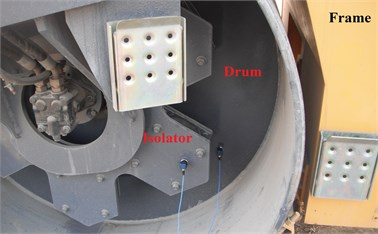a) Side view of drum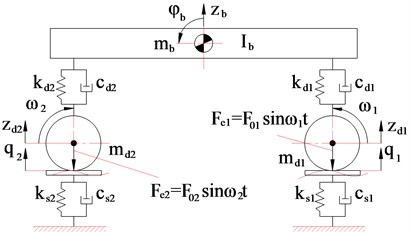b) Half -vehicle ride dynamic model

As shown in Fig. 1(b), ${m}_{d1}$, ${m}_{d2}$, and ${m}_{b}$ are the mass of the front drum, rear drum and vehicle body (including the frame, cab, and driver’s seat), respectively; ${k}_{d1}$, ${k}_{d2}$, and ${c}_{d1}$, ${c}_{d2}$ are the stiffness and damping coefficients of the isolation system of the front and rear drums, respectively; ${k}_{s1}$, ${k}_{s2}$, and ${c}_{s1}$, ${c}_{s2}$ are the stiffness and damping coefficients of the elastic soil ground contact with the front and rear drums, respectively; ${z}_{d1}$, ${z}_{d2}$, and ${z}_{b}$ are the vertical displacement of the front drum, rear drum and the vehicle body, respectively; ${\phi }_{b}$ is the pitch angle displacements of the vehicle body; ${I}_{b}$ is the moment of inertia with respect to the y of the vehicle body; ${q}_{1}$ and ${q}_{2}$ are the excitation of ground surface contact with the front and rear drums, ${F}_{1}={F}_{01}\mathrm{s}\mathrm{i}\mathrm{n}{\omega }_{1}t$ and .

The equations of motion for a double-drum vibrating roller using Newton’s second law of motion are written in two cases.

### 2.1. Case 1: vehicle moves into the workshop

Vehicle wheel contacts and moves on types of road or ground surfaces such as rigid, flexible, soft, etc. . The drum of vibratory roller in contact with the rigid road surface is the contact point which is considered in this study.

From the half-vehicle ride dynamic model, as shown in Fig. 1(b), the motion equations of the front vehicle body mass are written as follows:

1
${m}_{b}{\stackrel{¨}{z}}_{b}=-\left[{k}_{d1}\left({z}_{b}+{l}_{1}{\phi }_{b}-{q}_{1}\right)+{c}_{d1}\left({\stackrel{˙}{z}}_{b}+{l}_{1}{\stackrel{˙}{\phi }}_{b}-{\stackrel{˙}{q}}_{1}\right)\right]$
2
${I}_{b}{\stackrel{¨}{\phi }}_{b}=\left[{k}_{d2}\left({z}_{b}-{l}_{2}{\phi }_{b}-{q}_{2}\right)+{c}_{d2}\left({\stackrel{˙}{z}}_{b}-{l}_{2}{\stackrel{˙}{\phi }}_{b}-{\stackrel{˙}{q}}_{2}\right)\right]{l}_{2}$

where, $q$ is the random road surface excitation according to the International Standards Organization (ISO) 8608 .

### 2.2. Case 2: vehicle operates in the workshop

When vehicle works and compresses on many different types of properties of deformable ground soil such as the hysteretic force – deflection properties , the elasto-plastic properties , ., etc. The properties of deformable ground soil are linear by the stiffness and damping coefficients of the elastic soil ground. From the half -vehicle ride dynamic model as shown in Fig. 1(b), the motion equations of the front drum mass, rear drum mass and vehicle body mass are written as follows:

3
${m}_{d1}{\stackrel{¨}{z}}_{d1}={F}_{01}\mathrm{s}\mathrm{i}\mathrm{n}{\omega }_{1}t+\left[{k}_{d1}\left({z}_{b}+{l}_{1}{\phi }_{b}-{q}_{1}\right)+{c}_{d1}\left({\stackrel{˙}{z}}_{b}+{l}_{1}{\stackrel{˙}{\phi }}_{b}-{\stackrel{˙}{q}}_{1}\right)\right]-{k}_{s1}{z}_{d1}-{c}_{s1}{\stackrel{˙}{z}}_{d1},$
4
${m}_{d2}{\stackrel{¨}{z}}_{d2}={F}_{02}\mathrm{s}\mathrm{i}\mathrm{n}{\omega }_{2}t+\left[{k}_{d2}\left({z}_{b}-{l}_{2}{\phi }_{b}-{q}_{2}\right)+{c}_{d2}\left({\stackrel{˙}{z}}_{b}-{l}_{2}{\stackrel{˙}{\phi }}_{b}-{\stackrel{˙}{q}}_{2}\right)\right]-{k}_{s2}{z}_{d2}-{c}_{s2}{\stackrel{˙}{z}}_{d2},$
5
${m}_{b}{\stackrel{¨}{z}}_{b}=-\left[{k}_{d1}\left({z}_{b}+{l}_{1}{\phi }_{b}-{z}_{d1}\right)+{c}_{d1}\left({\stackrel{˙}{z}}_{b}+{l}_{1}{\stackrel{˙}{\phi }}_{b}-{\stackrel{˙}{z}}_{d1}\right)\right]$
6
${I}_{b}{\stackrel{¨}{\phi }}_{b}=\left[{k}_{d2}\left({z}_{b}-{l}_{2}{\phi }_{b}-{z}_{d2}\right)+{c}_{d2}\left({\stackrel{˙}{z}}_{b}-{l}_{2}{\stackrel{˙}{\phi }}_{b}-{\stackrel{˙}{z}}_{d2}\right)\right]{l}_{2}$

## 3. Optimal isolation parameters via genetic algorithm

Assessing the off-road vehicle’s ride comfort: There are four methods to objectively evaluate ride comfort such as the international standard ISO 2631, the British standard BS 6841, VDI 2057, and while average absorbed power (AAP) . The weighted root mean square (rms) of acceleration responses based on the international standard ISO 2631 is used as the objective function which is determined by the formula:

7

where $a\left(t\right)$ is the weighted acceleration (translational and rotational) as a function of time in m/s2; $T$ is the duration of the measurements in s.

The genetic algorithm is a technique adapted from the evolutionary adaptation of biological populations by Darwinism. GA is a method of random optimization by mimicking the evolution of humans or organisms. Its idea is to simulate natural phenomena inheriting and fighting for survival. A simple genetic algorithm consists of the following steps: Step 1: Initialize a population of chromosome sequences; Step 2: Determine the target value for each corresponding chromosome; Step 3: Create new chromosomes based on genetic operators; Step 4: Eliminate low adaptive chromosomes; Step 5: Define the target function for new chromosomes and population inclusion. Step 6: Check whether the stop condition is satisfied. If the condition is right, get the best chromosome, and the algorithm stops; otherwise, go back to step 3.

Objective function: To improve the vehicle’s ride comfort, the optimal parameters of drum’s isolation systems are found out so that the values of the weighted root mean square (rms) of acceleration responses of cab’s vertical vibration (${a}_{wz}$) and pitch vibration (${a}_{w\phi }$) should be minimized:

8
$F\left(X\right)={w}_{1}\left\{{a}_{wz}\left(X\right)\right\}+{w}_{2}\left\{{a}_{w\phi }\left(X\right)\right\}\to \mathrm{m}\mathrm{i}\mathrm{n},$

where, ${w}_{n}$ (1, 2) are the weighting coefficients of sub-objective functions such that (${w}_{1}+{w}_{2}=1$); $X=\left[{k}_{d1},{k}_{d2},{c}_{d1},{c}_{d2}\right]$ is the design parameter vector of drum’s isolation systems.

Boundary conditions: The objective function Eq. (8) must satisfy the following boundary conditions:

9

## 4. Results and discussion

In order to find out the values of the optimal parameters of drum’s isolation systems of a double-drum vibrating roller, the differential equations of motion in case 1 and case 2 are solved through Matlab/Simulink environment with the initial vehicle design parameters as shown in Table 1. A program of genetic algorithm is written in Matlab to declare input parameters such as objective function Eq. (8) with 0.75, 0.25, boundary conditions Eq. (9), and GA parameters such as population size as 50 and generation as 200, which called by Simulink module function using the sim function. When vehicle compacts on the original place with the parameters of the elastic soil grounds in Table 1 at both front and rear drums with the excitation frequencies of drums as 48 Hz (This condition is chosen for the optimal parameters because vehicle is often operates in this condition and it has the greatest impact on the drivers’ health), the optimal values of the parameters of drum’s isolation systems are obtained by GA method in comparison with the original parameters, as shown in Table 2.

Table 1Parameters of a double-drum vibrating roller

 Parameters Values Parameters Values Parameters Values ${m}_{d1}$ / kg 2300 ${k}_{d2}$ / (N/m) 1803×103 ${F}_{01}$ / N 128×103 ${m}_{d2}$ / kg 2300 ${c}_{d2}$ / (Ns/m) 3990 ${F}_{02}$/ N 96×103 ${m}_{b}$ / kg 3270 ${k}_{s1}$ / (N/m) 1×107 ${f}_{1}$ / Hz 48/54 ${I}_{b}$ / kg m2 7811 ${c}_{s1}$ / (Ns/m) 210×103 ${f}_{2}$/ Hz 48/54 ${k}_{d1}$ / (N/m) 1803x103 ${k}_{s2}$ / (N/m) 1.2×107 ${l}_{1}$ / m 1.80 ${c}_{d1}$ / (Ns/m) 3990 ${c}_{s2}$ / (Ns/m) 280×103 ${l}_{2}$/ m 1.80

Table 2GA optimization parameters of drum’s isolation systems

 Parameters ${k}_{d1}$ / (N/m) ${c}_{d1}$ / (Ns/m) ${k}_{d2}$ / (N/m) ${c}_{d2}$ / (Ns/m) Original values 1803×103 3990 1803×103 3990 Optimal values by GA 1017×103 2804 255×103 6130

The performances of the GA are compared with the original parameters of drum’s isolation systems when the vehicle operates at Case 2 with the excitation frequencies of drums as 48 Hz, as shown in Fig. 2.

From the results of Fig. 2, we can determine the values of the weighted rms acceleration of the vertical vehicle body (${a}_{wb}$) and pitch angle of vehicle body (${a}_{wphi}$) according to Eq. (7) are 0.6963 m/s2 and 0.0810 rad/s2 with the original parameters of drum’s isolation systems and 0.3497 m/s2 and 0.0604 rad/s2 with GA optimal parameters of drum’s isolation systems. The results show that the ${a}_{wb}$ and ${a}_{wphi}$ values with GA optimal parameters respectively reduce by 99.11 % and 34.11 % in comparison with the original parameters of drum’s isolation systems, which means that vehicle’s ride comfort is improved significantly when the vehicle operates in this condition.

The optimized results of GA are compared to those of the original parameters of drum’s isolation systems when the vehicle is operated at Case 2 with the different excitation frequencies of drums, as shown in Table 3. These results indicate that the ${a}_{wb}$ and ${a}_{wphi}$ values of GA optimal parameters respectively reduce by 87.89 % and 22.67 % (54 Hz and 54 Hz), 42.03 % and 42.65 % (0 and ${f}_{2}=$ 48 Hz), and 34.53 % and 34.92 % (0 and 54 Hz) in comparison with the original parameters of drum’s isolation systems, which means that the ride comfort of the double-drum vibrating roller is improved significantly.

Fig. 2The comparison of acceleration responses of vehicle body at Case 2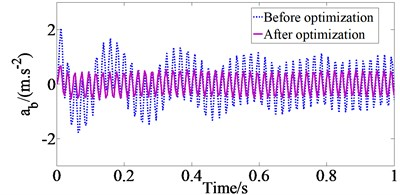a) Vertical vehicle body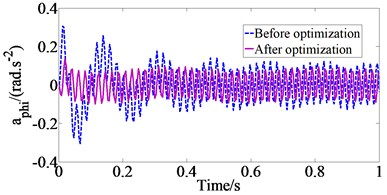b) Pitch angle of vehicle body

Table 3Comparison of optimized results at Case 2 with the different excitation frequencies

 Cases ${f}_{1}=$ 54 Hz and 54 Hz 0 and 48 Hz 0 and 54 Hz ${a}_{wz}$ (m.s-2) ${a}_{wphi}$ (rad.s-2) ${a}_{wz}$ (m.s-2) ${a}_{wphi}$ (rad.s-2) ${a}_{wz}$ (m.s-2) ${a}_{wphi}$ (rad.s-2) Original parameters 0.5723 0.0644 0.2984 0.2261 0.2466 0.1866 GA optimization 0.3046 0.0525 0.2101 0.1585 0.1833 0.1383 Decrease % 87.89 22.67 42.03 42.65 34.53 34.92

When the vehicle moves on the ISO class B road surface at the vehicle speed 15 km/h (Case 1), the acceleration responses of the vertical vehicle body (${a}_{b}$) and the pitch angle of the vehicle body (${a}_{phi}$) with GA optimal parameters in comparison with the original parameters of drum’s isolation systems are shown Fig. 3. From the results of Fig. 3, we can determine the ${a}_{wb}$ and ${a}_{wphi}$ values are 0.9822 m/s2 and 0.8142 rad/s2 with the original parameters and 0.1960 m/s2 and 0.1129 rad/s2 with GA optimal parameters of drum’s isolation systems. The results show that ${a}_{wb}$ and ${a}_{wphi}$ values with GA optimal parameters greatly reduce in comparison with the original parameters of drum’s isolation systems, which means that the performance optimization of drum’s isolation systems is better than the original isolation systems of drums in improving the ride comfort.

Fig. 3The comparison of acceleration responses of vehicle body at Case 1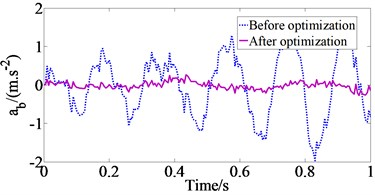a) Vertical vehicle body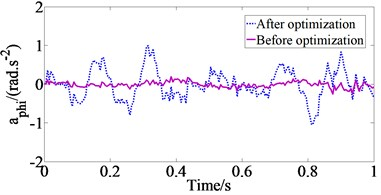b) Pitch angle of vehicle body

Table 4Comparison of optimized results at Case 1 with the different road surface conditions

 Cases ISO class C ISO class D ISO class E ${a}_{wz}$ (m.s-2) ${a}_{wphi}$ (rad.s-2) ${a}_{wz}$ (m.s-2) ${a}_{wphi}$ (rad.s-2) ${a}_{wz}$ (m.s-2) ${a}_{wphi}$ (rad.s-2) Original parameters 1.7673 1.4651 3.1023 2.5718 5.6108 4.6513 GA optimization 0.3528 0.2033 0.6194 0.3569 1.1203 0.6456 Decrease % 400.94 620.66 400.86 620.59 400.83 620.46

Similarly, the optimized results of GA are compared to those of the original parameters of drum’s isolation systems when the vehicle moves at the vehicle speed 15 km/h (Case 1) with the different road surface conditions, as shown in Table 4. These results indicate that the performance optimization of drum’s isolation systems has been greatly improved the vehicle ride comfort. However, GA optimal parameters have not been considered for the ride performance on the frequency domain.

## 5. Conclusions

In this study, a half-vehicle ride dynamic model of a double-drum vibrating roller is established under different operating conditions for searching the optimal parameters for drum’s isolation systems to improve the ride comfort. A genetic algorithm is applied for searching the optimal parameters of drum’s isolation system order to minimize the ${a}_{wz}$ and pitch ${a}_{w\phi }$ values in order to achieve the best comfort of a double-drum vibrating roller. The study results show that the ${a}_{wb}$ and ${a}_{wphi}$ values with GA optimal parameters greatly reduce in comparison with the original parameters of drum’s isolation systems, which means that vehicle’s ride comfort is improved significantly. Also, the study results can provide the theoretical basis for the isolation system design of the vibrating rollers.

#### Cited by

Advances in Engineering Research and Application
Advances in Engineering Research and Application
Advances in Engineering Research and Application
Advances in Engineering Research and Application
Improvement of Ride Comfort Quality for an Earth-Moving machinery with Semi-Active Cab Isolation System
Optimal design parameters of air suspension systems for semi-trailer truck. Part 2: results and discussion

27 April 2020
Accepted
04 May 2020
Published
07 May 2020
SUBJECTS
Vibration in transportation engineering
Keywords
vibratory roller
drum
isolation system
design parameter
genetic algorithm
ride comfort